# Bits, Bytes and Beers

If you’re reading this you are undoubtedly a computer musician or audio engineer and likewise you probably have a general understanding of the bits and bytes of the binary world. But for many, simply knowing that 24 bit is better than 16-bit, but not being able to coherently explain why, can be frustrating.

Why is the MIDI range 0 – 127? What is a 14 bit controller? If you’re a programmer or already fluent in binary expressions, stop here and grab yourself a beer. But if not and you’re curious to know what’s going on underneath the hood of your CPU, read on.

### Basics

1 bit stores either 0 or 1. Two choices and hence the term binary.
1 byte is a grouping of 8 bits.

The more bits you use to define a quantity, the more accurate you can be, simply because there are more choices. As an analogy think of a pitch control. If you can only adjust the pitch by half-steps, that gives you twelve possible values within each octave. But a fine pitch control will often allow 100 cents or choices per step, which would result in 1200 possible choices.

Another analogy is digital color. Below is an example of an 8 bit color palette. There are 256 possible choices. Below that is a 24 bit color spectrum which allows millions of choices.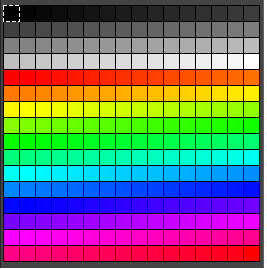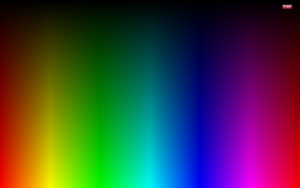Still confused? Let’s consider the beer analogy. Suppose you walk into a bar and they have just 4 beers on tap. That’s a 2 bit beer choice. Now suppose they have 256 beers on tap. That’s an 8 bit beer choice. Conclusion: 8 bits is better than 2 bits. Still confused? So am I, but read on.

## Binary vs. Base 10

To understand the binary system, it would be useful to understand how base 10 numbers correspond to binary numbers.

In a base 10 system each place in a decimal number is represented by 10 possible values (0 – 9). For example, the expression 12,457 is equivalent to the sum of the values below:

1 x 10,000 = 10,000
2 x 1,000 = 2000
4 x 100 = 400
5 x 10 = 50
7 x 1 = 7

7 + 50 + 400 + 2000 + 10,000 = 12,457.

In a binary system, each place can only have 2 possible values (0 or 1). Each successive place is a power of 2. So for an 8 bit number you would have a group of 8 digits, all either 0 or 1.

Note the table below:
1 = 20
2 = 21
4 = 22
8 = 23
16 = 24
32 = 25
64 = 26
128 = 27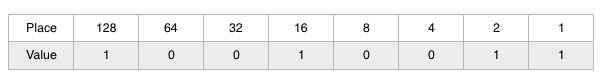As an example, I’ve assigned random values to each place above. So the binary value 10010011 in base-10 would equal 147:

1 x 1 = 1
1 x 2 = 2
4 x 0 = 0
8 x 0 = 0
16 x 1 = 16
32 x 0 = 0
64 x 0 = 0
128 x 1= 128

1 + 2 + 16 + 128 = 147

If the case of an 8 bit binary number, the max value would be 255 (with each place value being 1):

1 + 2 + 4 + 8 + 16 + 32 + 64 + 128 = 255

But there would actually be 256 possible values because 0 is counted as a value (0 – 255).

To determine the total number of possible values in a binary system you could simply raise 2 by the power equal to the number of bits.

For example, let’s consider 16 bit audio at a sampling rate of 44.1k (audio CD specifications). In this case, 44,100 samples or measurements are taken every second. Each sample can have 1 of a possible 2^16 (2 raised by an exponent of 16) values or 65,536. For 24 bit audio at the same sampling rate, each sample can have 1 of a possible 2^24 (2 raised by an exponent of 24) values or 16,777,216. So you can see that 24 bit audio can be much more specific in terms of a digital value. More specificity means more accuracy and less noise or error in describing an analog source. In terms of dynamic range, this translates to 140dB for 24 bit recording vs. just 96 dB for 16 bit recording.

## MIDI

What about MIDI? What’s with this 0 – 127 range? Going back to the 8 bit chart above you should begin to see where that’s coming from.

ADVERTISEMENT

Typical MIDI Values are derived from 7 bit binary numbers: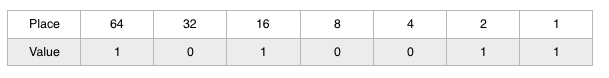So the 7 bit binary value above, 1010011 in base 10 would equal 83:

1 x 1 = 1
2 x 1 = 2
4 x 0 = 0
8 x 0 = 0
16 x 1 = 16
32 x 0 = 0
64 x 1 = 64

1 + 2 + 16 + 64 = 83

If all places had value of 1 we would have 64 + 32 + 16 + 8 + 4 + 2 + 1 = 127.
If all places had value of 0 we would have 0.
So the range is 0 to 127, which equals 128 possible values.

Certain MIDI controllers can be set for finer control up to 14 bit. In that case, you could have 2^14 (2 raised to an exponent of 14) or 16,384 possible values instead of just 128 (often useful for pitch bend control).

## 1 Bit — High Sampling Rate Recording

In terms of digital recording, there have been other approaches. Sony/Philips developed the 1 bit recording system known as DSD (Direct Stream Digital) that uses extreme sampling rates (2.8224MHz). The Korg MR-1000 uses a sample rate of 5.6448MHz. In these cases, accuracy is achieved via the sheer number of sequential measurements or samples, versus fewer but more specific measurements.

## 32 and 64 bit Architecture

CPUs can be described as using 32 bit or 64 bit architecture. The transformation to 64 bit started around 2006, but it took a few years for software and operating systems to catch up. To take full advantage of 64 bit, each component in the chain has to be operating in that world (e.g. application, operating system, and CPU).

Benefits of 64 bit architecture:

• Increased Precision
• Increased Dynamic Range
• Increased Headroom

Good article on the differences between 32 and 64 bit here.

## Floating Point vs. Fixed Point

Below are two numbers of equal value:

2,326,000,000,000,000,000.0 = 2.326 x 10^18 (10 raised by an exponent of 18)

Note that it takes 19 digits to represent the Fixed Point number on the left, while it only takes 8 digits to represent the same value using a Floating Point number on the right. The same idea can be used to express very small numbers by using a negative exponent.

Without getting entrenched in the math, suffice it to say that a fixed point number can be more precise, but a floating point number can express very large or very small numbers using less bits. In terms of audio, this translates to increased headroom and dynamic range for float point processing, but potentially less precision and more rounding errors. This means that a file recorded at 32 bit float may actually have more errors than one recorded at 24 bit fixed. Indeed, even with DAWs that allow for a 32 bit float point session preference, files are actually recorded at 24 bit, and then converted internally before saving to a file.

After the sound is recorded at 24 bit, any processing including EQ or any added effects, and even the simple mixing of non manipulated tracks is done at the highest bit resolution available to the CPU, operating system, application and plugin.

A good discussion on the subject can be found here (page 65-78):

So the next time someone asks you questions like why 24 bit recording is better than 16 bit or why the MIDI range is 0-127, hopefully you’ll have an answer that sounds coherent. If not, mumble something under your breath and offer them a beer.

NOTE: In this article, if it wasn’t obvious, beer is used as a metaphor for your own flavor of personal reward. That being said, if you do drink, drink responsibly. And if you don’t, that’s more money for gear!

### Missing our best stuff?

Sign up to be the first to learn about the latest articles, videos, courses, freebies, giveaways, exclusive discounts and more.

We'll never spam you. Unsubscribe at any time. Powered by ConvertKitPhilip Mantione is a composer, synthesist, guitarist, educator and sound artist active in the LA experimental music scene. His music has been presented in festivals, museums and galleries worldwide. His current project is TriAngular Bent, an electroacoustic trio featuring Don Preston (founding member of Frank Zappa’s Mothers of Invention) and circuit bending virtuoso, Jeff Boynton. Details at philipmantione.com

### 3 FREE Max for Live Devices

Download three free Max for Live Devices from Phil Mantione.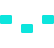/>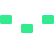/>/>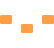/>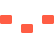/>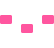/>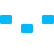/>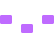/>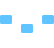/>/>